# The Math behind Manholes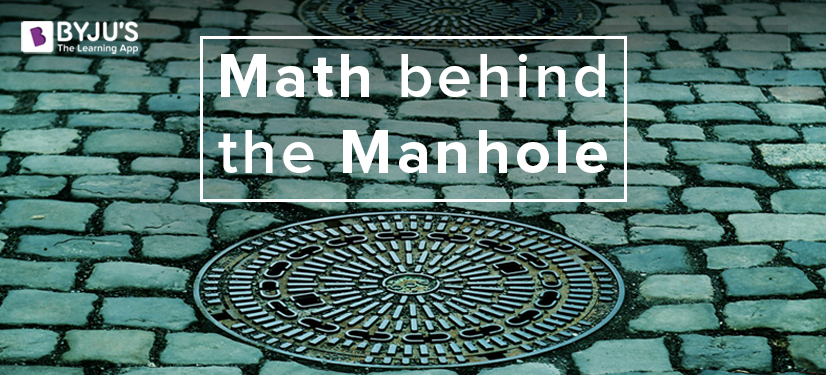They are found in every city, on every road – they are everywhere! Whether the issue is with sewers, electricity or telephone lines, they come to our rescue. They are mostly round in shape. They serve as an excellent hiding spot to Dr. Curt Connors (The Lizard) as Peter Parker (Spiderman) chases him. Well, you might have guessed what we are talking about, by now. They are ‘manholes’. And if you still can’t recall them, here (see fig. 1) is an image of a typical manhole (The word ‘DANGER’ should warn you not to play around one of those).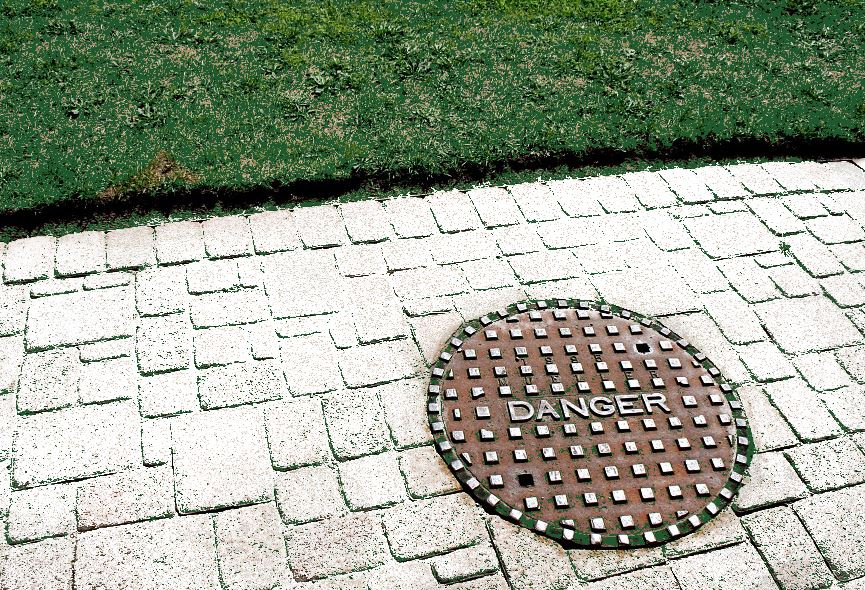Figure 1: A typical manhole

But why are most manhole covers round in shape? When we asked the same question in a class full of students, we got two answers. One, they can be easily rolled and two, placing them in position is easy in any alignment. But as it turns out, a round manhole cover, a circle (mathematically speaking) has another very interesting property.

To understand why most of the manholes are round and not square or triangular, you will have to tinker with a little bit of math. Let us perform an experiment. Take a square and place it between two parallel lines (see fig. 2). If you rotate the square, the lines move apart from each other and then come back together (see fig. 3).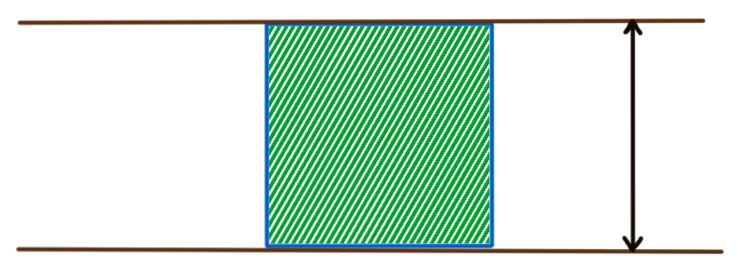Figure 2: Square placed between two parallel lines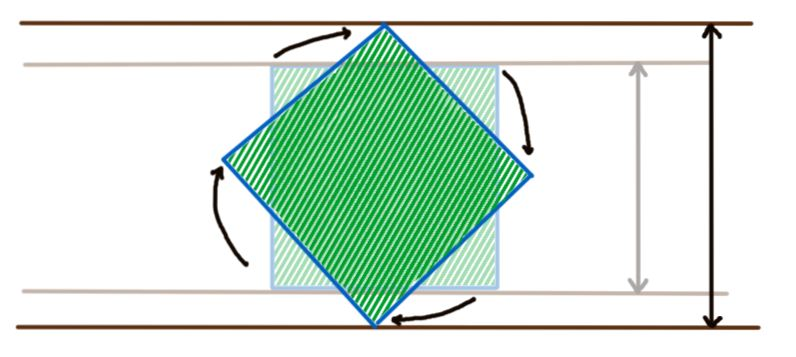Figure 3: When square rotates, distance between the lines changes

But if we keep a circle between two lines and rotate it, the distance between the lines will remain fixed (see fig. 4). This peculiar nature makes a circle a curve of constant width. At this point, you may ask if there are any other shapes which have a constant width. Well, there are. Math hardly disappoints!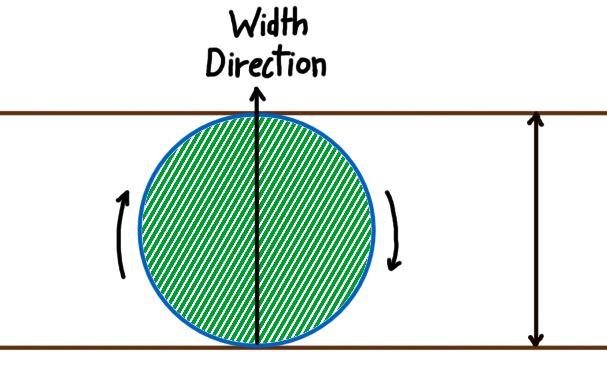Figure 4: Circle as a curve of constant width

The width of the curve in a given direction is defined as the distance between two supporting lines perpendicular to that direction.

What’s a supporting line?

A supporting line is defined as a straight line which has at least one point in common with the curve it is supporting and the curve should lie on any one side of the line. In fig. 4, both the lines are hence supporting lines.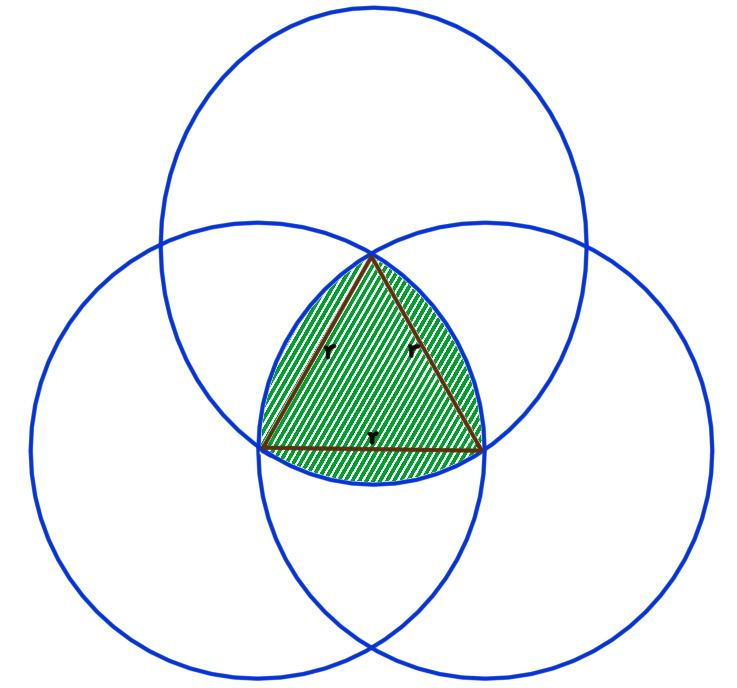Figure 5: Construction of a curve of constant width

Now, cut along the perimeter of the shaded region in fig. 5. The curve so obtained (see fig. 6) is known as Reuleaux triangle (pronounced as Roo-Low triangle). It is named after Franz Reuleaux (1829 – 1905), a German mechanical engineer.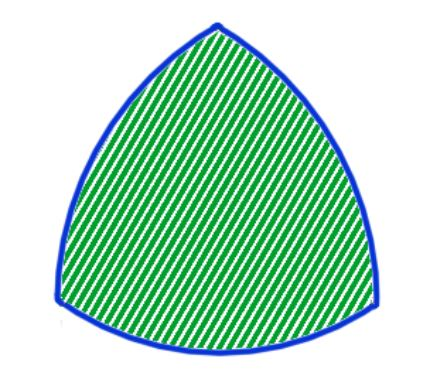Figure 6: Reuleaux Triangle

Reuleaux triangle is a curve of constant width. It would hence rotate keeping the distance between the lines constant (see fig. 7).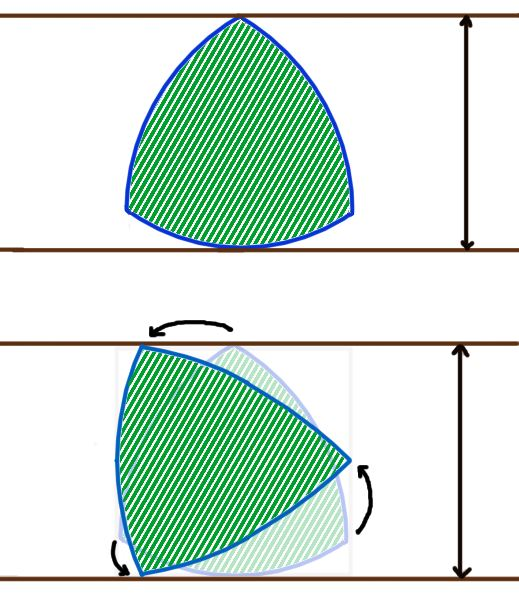Figure 7: Illustration of Reuleaux triangle as a curve of constant width

A Reuleaux polygon can be constructed for any regular polygon with an odd number of sides.

One interesting fact is if you roll one Reuleaux polygon along the boundary of another, the shape traced by the center of your polygon will give another Reuleaux polygon. Some of the Reuleaux polygons are shown in fig. 8.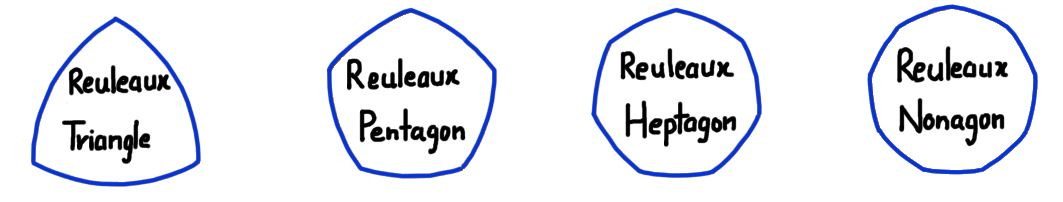Figure 8: Reuleaux polygons

The perimeter of any Reuleaux polygon can be given by this formula:

Perimeter = Π × Diameter

where the diameter is the width of the Reuleaux polygon. This was first proved by Joseph-Émile Barbier (1839–1889), who was a French astronomer and mathematician, in 1860. After him, the above theorem is called Barbier’s theorem.

So, this formula for the perimeter is not something that is exclusive to a circle. Any two or more Reuleaux polygons having the same width will hence have the same perimeter (see fig. 9).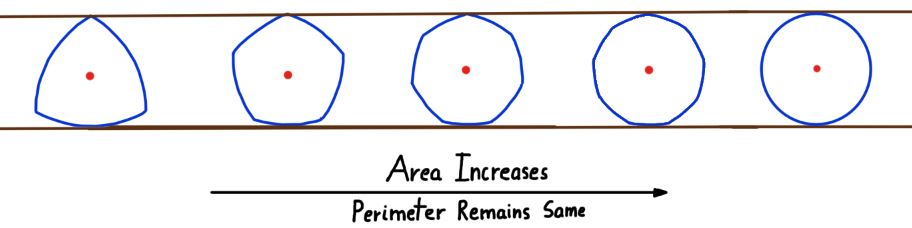Figure 9: Illustration of Barbier’s theorem

Hence, if all the above Reuleaux polygons have the same width, the triangle will have the least area and the circle will have the largest.

Suppose you made a wheel in the shape of Reuleaux triangle. It will cover the exactly the same distance in one rotation as a circular wheel of the same width. On top of that it will spare you some material required to make the wheel since the area is less than that of a circle.

The obvious question here is why don’t we use Reuleaux triangle for making wheels then? It is because its center doesn’t remain stationary like that of the circle when it rotates unlike circle.

It moves in a nearly circular path as the Reuleaux triangle rotates. This would result in bumps which would make you uncomfortable. But it is used at other places such as drilling square holes because when it rotates, its vertices trace a square (with round corners) shape.

If you are thinking that this concept exists only in a two-dimensional world, you would probably be surprised to see that it’s not! If you rotate a Reuleaux triangle along its central line, the shape obtained is called Reuleaux tetrahedron (see fig. 10). Just like the sides of a Reuleaux triangle were curved, so are the surfaces of the Reuleaux tetrahedron.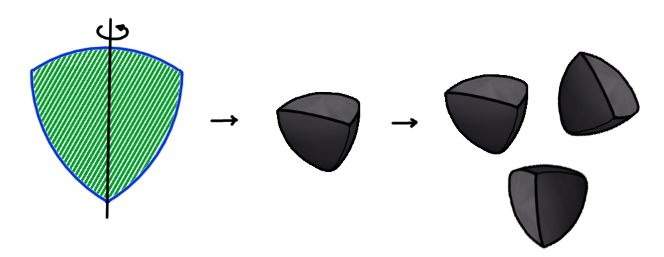Figure 10: Reuleaux Polyhedrons

Now, what you are about to hear, may blow your mind. If you lay marbles of the shape of Reuleaux polyhedrons on a smooth surface and skate on them, you will feel exactly as if they were spherical marbles. Reuleaux polyhedron keeps two planes separated by the same distance just like Reuleaux triangle kept two lines separated by a constant distance. What about four- dimensional Reuleaux objects? Are they possible? Yes? No? May be? We aren’t sure of that but one thing that we do know is that Math is limitless.

Let us get back to manholes now. If they are of any shape which is not of constant width (such as square), the lid may line up with the wider part of the hole and fall inside. But a curve of constant width will never fall inside. So, if you ever want to explore a square manhole, make sure it is hinged before going inside!

J.md.hamza

October 9, 2018

I am interested in the byjus

Testimonials

### Book Your Free Class Now